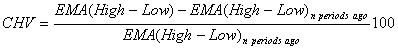Chart Pro online
User name (email):

 Recently used

 Forex

 Indices

# Chaikin Volatility (CHV)

Chaikin Volatility (CHV) is indicator in technical analysis which determines volatility of financial instrument using percent change in a moving average of subtraction of high and low over some period of time.

Chaikin volatility is calculated by formula:For calculation, CHV uses exponential moving average EMA. For EMA period value of 10 is usually used. Same value is used for parameter n.Chaikin Volatility (CHV)

Interpretation

If value of CHV is increased in short period of time, it could be indication of market bottom. On the other hand, if value of CHV is decreased in short period of time, it could be indication of market top.

Example